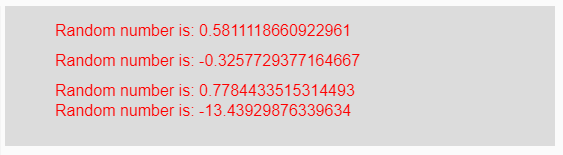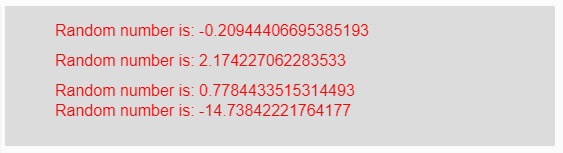# p5.js | randomGaussian() Function

The randomGaussian() function in p5.js is used to return a random value fitting a Gaussian or normal distribution for which mean and standard deviation are given as the parameter.

Syntax:

`randomGaussian( Mean, StandardDeviation )`

Parameters: This function accepts two parameters as mentioned above and described below:

• Mean: It is the mean of generated random value.
• StandardDeviation: It is the Standard Deviation of generated random value.

Note:

• If one argument is passed as the parameter it means that the mean and standard deviation is 1.
• If two argument is passed as the parameter it means that first is mean and second is a standard deviation.
• If no argument is passed as the parameter it means that mean is 0 and the standard deviation is 1.

Return Value: It returns a random number.

Below programs illustrate the randomGaussain() function in p5.js:

Example 1: This example uses randomGaussian() function return the random value for which mean and standard deviation is given as the parameter.

 `function` `setup() {  ` `   `  `    ``// Creating Canvas size ` `    ``createCanvas(550, 140);  ` `       `  `    ``// Set the background color  ` `    ``background(220);  ` `    `  `    ``// Calling to randomSeed() function ` `    ``// It is used for getting constant random ` `    ``// values each time the code is run ` `    ``randomSeed(9) ` `      `  `    ``// Calling to randomGaussian() function ` `    ``// with mean and sd parameters ` `    ``let A = randomGaussian(1, 2); ` `    ``let B = randomGaussian(0, 1); ` `    ``let C = randomGaussian(2); ` `    ``let D = randomGaussian(2, 10); ` `      `  `    ``// Set the size of text  ` `    ``textSize(16);  ` `       `  `    ``// Set the text color  ` `    ``fill(color(``'red'``));  ` `     `  `    ``// Getting random number ` `    ``text(``"Random number is: "` `+ A, 50, 30); ` `    ``text(``"Random number is: "` `+ B, 50, 60); ` `    ``text(``"Random number is: "` `+ C, 50, 90); ` `    ``text(``"Random number is: "` `+ D, 50, 110); ` `} `

Output:Note: In the above example, the variable “C” contains one parameter i.e. mean value is 2 but the standard deviation is 1.

Example 2: This example uses randomGaussian() function return the random value for which mean and standard deviation is given as the parameter.

 `function` `setup() {  ` `  `  `    ``// Creating Canvas size ` `    ``createCanvas(550, 140);  ` `      `  `    ``// Set the background color  ` `    ``background(220);  ` `   `  `    ``// Calling to randomSeed() function ` `    ``// It is used for getting constant random ` `    ``// values each time the code is run ` `    ``randomSeed(9) ` `     `  `    ``// Calling to randomGaussian() function with ` `    ``// mean and sd parameters ` `    ``let A = randomGaussian(); ` `    ``let B = randomGaussian(2.5); ` `    ``let C = randomGaussian(2); ` `    ``let D = randomGaussian(20, 22.5); ` `     `  `    ``// Set the size of text  ` `    ``textSize(16);  ` `      `  `    ``// Set the text color  ` `    ``fill(color(``'red'``));  ` `    `  `    ``// Getting random number ` `    ``text(``"Random number is: "` `+ A, 50, 30); ` `    ``text(``"Random number is: "` `+ B, 50, 60); ` `    ``text(``"Random number is: "` `+ C, 50, 90); ` `    ``text(``"Random number is: "` `+ D, 50, 110); ` `}  `

Output:My Personal Notes arrow_drop_upCheck out this Author's contributed articles.

If you like GeeksforGeeks and would like to contribute, you can also write an article using contribute.geeksforgeeks.org or mail your article to contribute@geeksforgeeks.org. See your article appearing on the GeeksforGeeks main page and help other Geeks.

Please Improve this article if you find anything incorrect by clicking on the "Improve Article" button below.

Article Tags :

Be the First to upvote.

Please write to us at contribute@geeksforgeeks.org to report any issue with the above content.